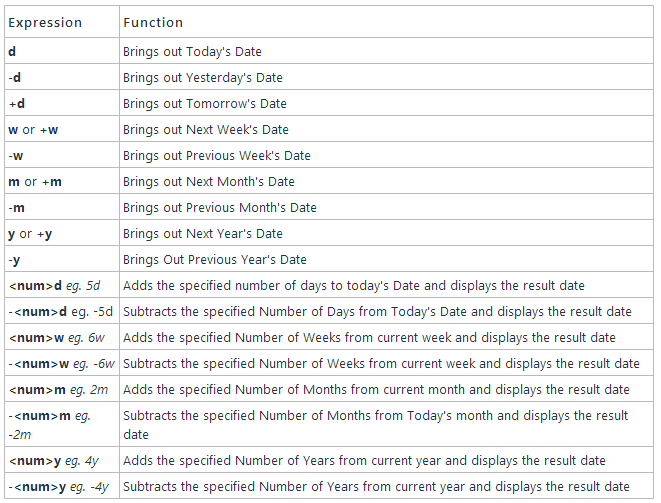# Fun with Date Expressions

Much emphasis has been given to make sure that MixERP comes out with one of the most elegant user interface for an ERP application. But while doing so, we also make sure that the functionalities of a standard ERP applications are not compromised. Hence, MixERP has been embedded with some very powerful tools and features under the surface. The date expression is among one such example that has been fully implemented into the application already. The Date Expressions in MixERP help you quickly enter corresponding dates into the application by entering simple alphabetical expressions. This will help you save time since you will not be required to type-in/select the entire date every time. For example if you want to enter today's date simply type "d" and hit TAB or click on any other field, MixERP will automatically enter today's date. If you want to enter yesterday's date just type in "-d". Similarly, enter +d for tomorrow's date or "2d" if you want to enter the date of the day after tomorrow. List of all Expressions Applicable  Please Note that these expressions are applicable only for the Date Fields inside the application.Now for those of you who want to get to the nitty gritty of things, here is the script behind:

``````\$(document).ready(function() {
\$(".date").blur(function() {
if (today == "") return;
var control = \$(this);
var value = control.val().trim().toLowerCase();
var result;

if (value == "d") {
result = dateAdd(today, "d", 0);
control.val(result);
Page_ClientValidate(control.attr("id"));
return;
}

if (value == "m" || value == "+m") {
control.val(dateAdd(today, "m", 1));
Page_ClientValidate(control.attr("id"));
return;
}

if (value == "w" || value == "+w") {
control.val(dateAdd(today, "d", 7));
Page_ClientValidate(control.attr("id"));
return;
}

if (value == "y" || value == "+y") {
control.val(dateAdd(today, "y", 1));
Page_ClientValidate(control.attr("id"));
return;
}

if (value == "-d") {
control.val(dateAdd(today, "d", -1));
Page_ClientValidate(control.attr("id"));
return;
}

if (value == "+d") {
control.val(dateAdd(today, "d", 1));
Page_ClientValidate(control.attr("id"));
return;
}

if (value == "-w") {
control.val(dateAdd(today, "d", -7));
Page_ClientValidate(control.attr("id"));
return;
}

if (value == "-m") {
control.val(dateAdd(today, "m", -1));
Page_ClientValidate(control.attr("id"));
return;
}

if (value == "-y") {
control.val(dateAdd(today, "y", -1));
Page_ClientValidate(control.attr("id"));
return;
}

if (value.indexOf("d") & gt; = 0) {
var number = parseInt(value.replace("d"));
control.val(dateAdd(today, "d", number));
Page_ClientValidate(control.attr("id"));
return;
}

if (value.indexOf("w") & gt; = 0) {
var number = parseInt(value.replace("w"));
control.val(dateAdd(today, "d", number * 7));
Page_ClientValidate(control.attr("id"));
return;
}

if (value.indexOf("m") & gt; = 0) {
var number = parseInt(value.replace("m"));
control.val(dateAdd(today, "m", number));
Page_ClientValidate(control.attr("id"));
return;
}

if (value.indexOf("y") & gt; = 0) {
var number = parseInt(value.replace("y"));
control.val(dateAdd(today, "y", number));
Page_ClientValidate(control.attr("id"));
return;
}
});
});

function dateAdd(dt, expression, number) {
var d = Date.parseExact(dt, shortDateFormat);
var ret;

if (expression == "d") {
ret = new Date(d.getFullYear(), d.getMonth(), d.getDate() + parseInt(number));
}

if (expression == "m") {
ret = new Date(d.getFullYear(), d.getMonth() + parseInt(number), d.getDate());
}

if (expression == "y") {
ret = new Date(d.getFullYear() + parseInt(number), d.getMonth(), d.getDate());
}

return ret.toString(shortDateFormat);
}``````2013-10-28T14:15:00-04:00